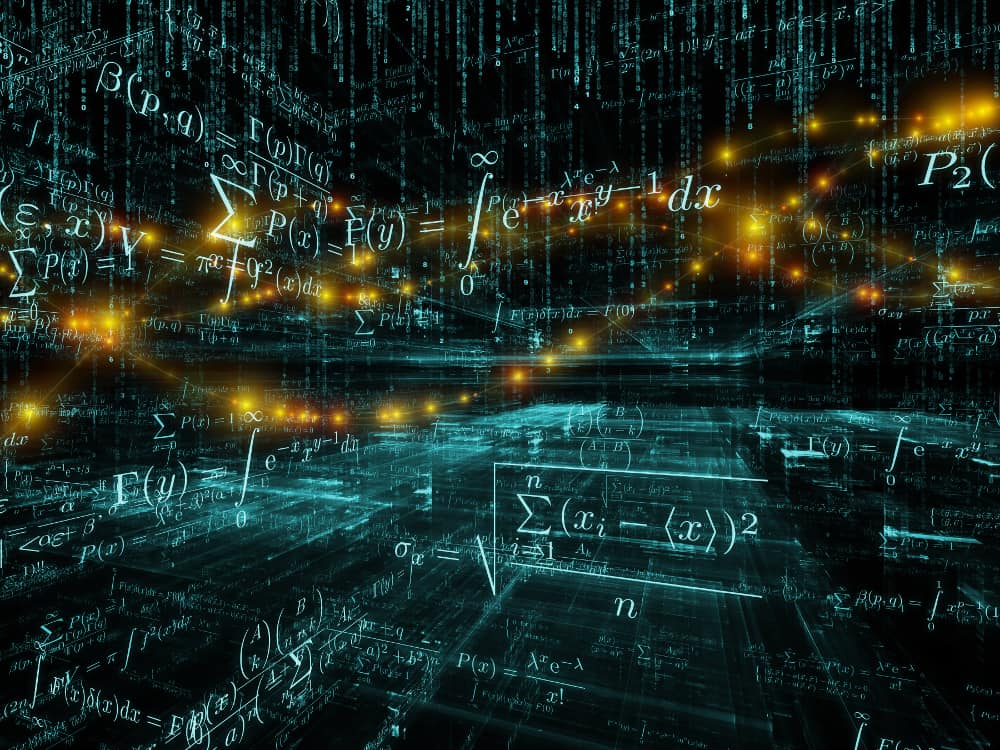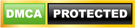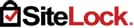## Artificial Intelligence Mathematical Formula Developer and SolverThis invention concept pertains to a machine learning system that automatically develops and learns new forms of mathematics, such as around theories like particle physics and quantum mechanics, as well as spatial anomalies and other rare instances. The system would essentially learn all existing forms of math, and then develop it’s own form of mathematical languages, which would yield large amounts of mathematical formulas, that are computer based, that can explain simple to complex problems and systems that are either inputted as pictures, videos, text, or other forms of formatted data that the system can understand and study based on various types of known mathematics like statistics, and then expand on the studies with unique forms of mathematics that the system develops to explain even more granular details of occurrences within the data. The system, ideally, would be based on a quantum computer with at least 150 Q-bits of processing power, as the mathematical details would be limited on computers with less processing power due to the limitations of calculating prime numbers and other types of patterns that can be used to study uniqueness.